# SPSS AMOS Mediation Analysis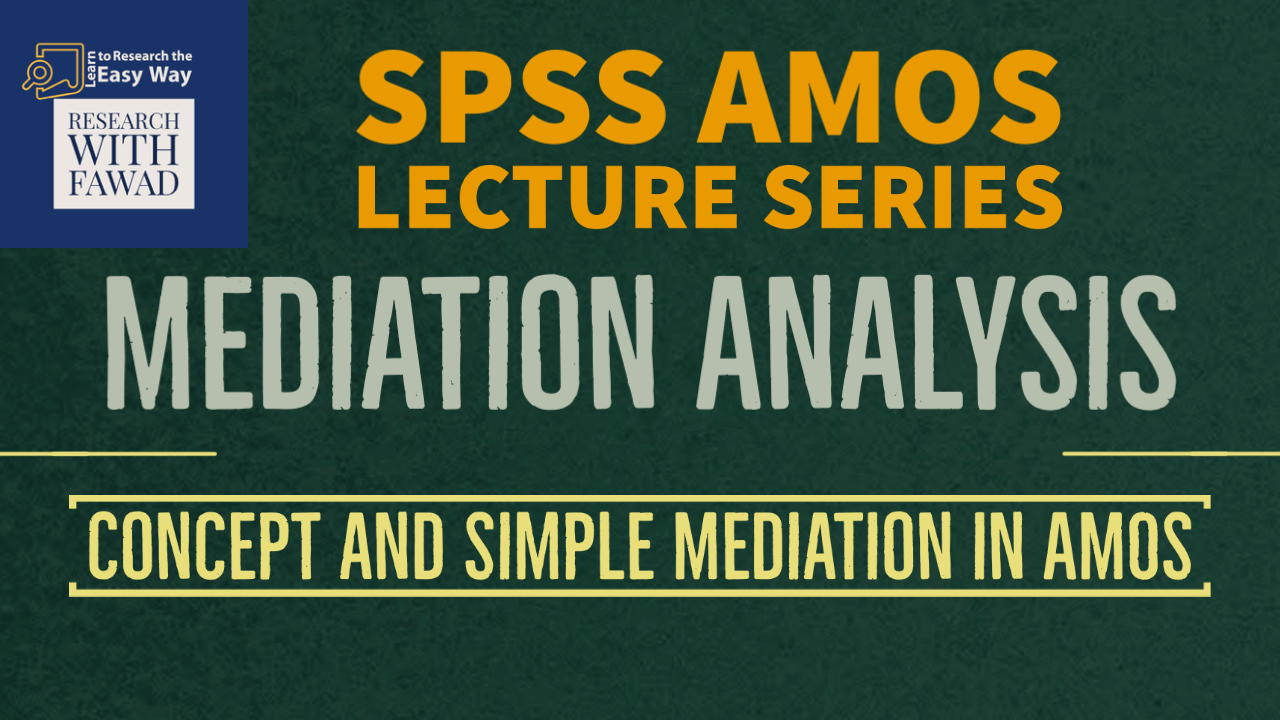### Mediation Analysis in SPSS AMOS

The Focus of the video tutorial is guiding how to designing, interpreting, and reporting a full structural model in SPSS AMOS.

## Introduction to Mediation Analysis

Up to this point, we have focused on how one construct can directly influence another construct in a SEM model.

• Let’s now examine how the influence between two constructs may take an indirect path through a third variable called a mediator.
• In these situations, the third variable will intervene on the influence of the two constructs (Hair et al. 2009).
• In testing if “mediation” or the presence of a mediator in a model, you need to understand some of the terminology that is used, such as direct effect, indirect effect, and total effects.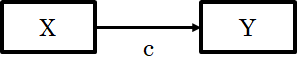• c in the relationship between X and Y is the total effect.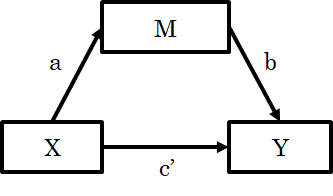• A direct effect is simply a direct relationship between an independent variable and a dependent variable in presence of the Mediator (c’).

• An indirect effect is a relationship that flows from an independent variable to a mediator and then to a dependent variable (a*b).

• The term total effect is the combined influence of the direct effect between two constructs and the indirect effect flowing through the mediator (c = c’ + a*b).

## Forms of Mediation

• Mediation can take numerous forms in a model. You can have what is called full mediation (also called indirect only mediation) where the direct effect between two constructs is non-significant, but an indirect effect through a mediator does have a significant relationship.
• Partial mediation is another form that mediation can take. This is where the direct effect between two constructs is significant, and so is the indirect effect through a mediator.

Lastly, you can have complementary and competitive mediation.

• Complementary mediation is where the direct effect and the indirect effect have a similar influence in regard to directionality. For instance, the direct effect may a have positive influence, and the indirect effect has a positive influence as well.
• Competitive mediation is where you have different directionality between the direct effect and indirect effect. The direct effect might have a negative influence, but the indirect effect might have a positive influence. With this type of mediation, the presence of the mediator can change the directionality of the influence.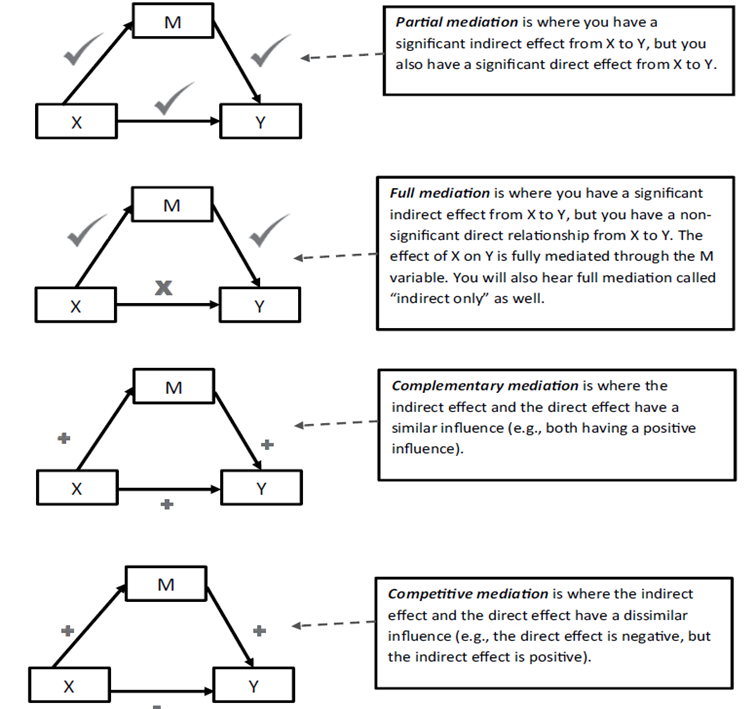## A Simple Mediation Model

• Let’s look at a simple mediation model to give some context to our discussion.
• We have an independent variable (we will label it “X”) that has a proposed direct influence on a dependent variable (we will call it “Y”). We will also propose that the influence of X to Y might flow through a mediator variable (we will call it “M”). We have three variables in this simple model.
• We are going to examine the direct effect of X to Y and also the indirect effect of X to M to Y.
• From a statistical standpoint, you will often see the paths in a mediation model referred to as the “A path”, the “B path”, and the “C path”. The commonly referred-to A path is the relationship from the independent variable to the mediator (X to M).
• The B path is used to refer to the relationship from the mediator to the dependent variable (M to Y). The C path is used when referring to the direct path from the independent variable to the dependent variable (X to Y).
• The indirect effect is calculated by taking the product of the X to M (a) relationship and the M to Y (b) relationship. We will simply multiply the regression coefficients for each of those relationships to get the indirect effect (a*b).
• This is common vernacular when discussing mediation, and you need to take note of these parameter labels. To help clarify our discussion, the different types of mediation are represented in graphical form. See Example.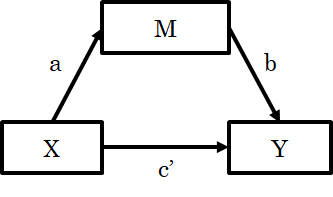## How to Test Mediation - The Old Approach

• The research by Baron and Kenny (1986) was one of the fundamental frameworks for how to test mediation. Over the years, research has refined their initial work on testing mediation.
• I think it is a worthy pursuit to discuss where mediation testing started and where it has progressed today. Baron and Kenny (1986) stated there were four steps to testing mediation:
• Step 1—make sure that X has a significant influence on Y (C path; absent of M at this point).
• Step 2—test that X has a significant influence on M (A path; no Y included); this needs to be significant for mediation to be present.
• Step 3—test that X has an influence on M and that M has an influence on Y (A and B paths); both paths need to be significant.
• Step 4—test the direct and indirect relationships simultaneously and determine if and what type of indirect effect is present (A, B, and C paths are all being evaluated).

## Criticism on Baron and Kenny Approach

• The Baron and Kenny method was based on finding the unstandardized coefficients for each relationship and then determining significance using a Sobel test.
• As research has progressed, this method of testing mediation has changed, and Sobel testing has been rejected as a valid means of testing mediation.
• Even the initial steps outlined by Baron and Kenny have changed as well. The first step that the C path needs to be significant is not a requirement anymore. Indirect effects can be present even if a non-significant C path is initially found.
• The justification is based on the idea that there are suppressor effects that prevent the C path from being significant, but the indirect effect is still present.
• The idea that the A path and the B path have to individually be significant has been rejected as well. Hayes (2018) notes that an indirect effect is the product of the A and B paths, and statistical significance of either the A path or the B path is not a requirement for mediation.

## Revised Method for Mediation Analysis

• The revised method is now concerned with assessing the indirect effect by examining the product of the A path and the B path while controlling for the direct effect of the C path.
• Since the Sobel test is flawed for this type of test, the more accepted approach in mediation testing is to use a bootstrap technique to determine significance.
• A bootstrap technique treats your data sample like a pseudo-population and then takes a random sample with replacement to determine if your indirect effect falls within a confidence interval.
• You can request the number of bootstrap samples to increase the accuracy of predictions (the higher, the better). A bootstrap sample of 5,000 is sufficiently large, and any greater number of samples will produce very little difference.
• Note that with a bootstrap sample, the computer program will generate a completely different sample every time you run the analysis. With 5,000 samples, the differences will be small, but the exact numbers in the results will not be the same if you run the analysis twice.
• To control for this, you can ask AMOS to always use the same “seed” number, which will produce the exact same results if you run the bootstrap analysis again. I will look into seed number in later videos

## Example Model

• Let’s look at an example in AMOS of a mediation test. Using the full structural model example, we want to examine if the construct of Authentic Leadership has an indirect effect through Self-Efficacy to the construct of Life Satisfaction.
• Notice that I am including a direct path from Authentic Leadership to Life Satisfaction. This will allow to see what type of mediation is present in the analysis.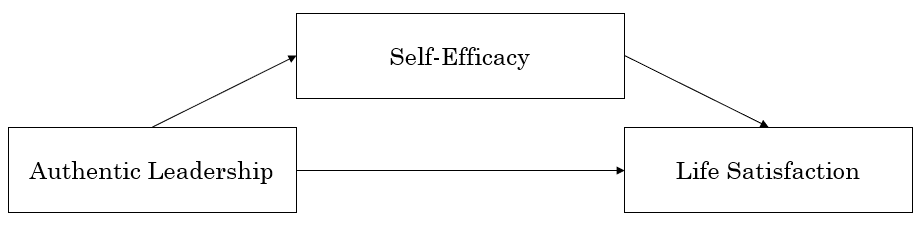## Step 1: Analysis Properties

• To determine if the indirect effect of Authentic Leadership to Life Satisfaction is significant, we need to request from AMOS the indirect, direct, and total effects in the output. This will give all possible indirect effects in the model.
• To do this, select the Analysis Properties button , and when the Analysis Properties pop-up window appears, go to the Output tab at the top. On that tab, you will see at the top right an option for “Indirect, direct, and total effects”. Select this option.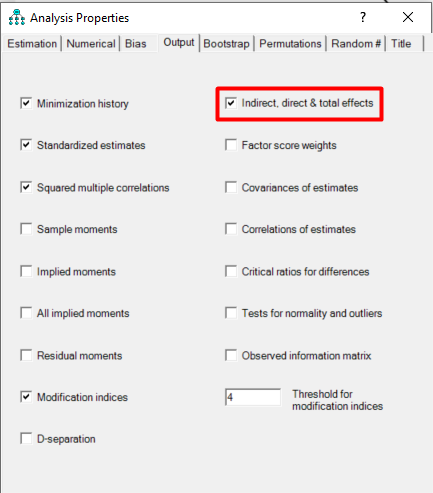## Step 2: Bootstrap Properties

• Next, we need to request a bootstrap analysis in AMOS. To do this, go to the bootstrap tab at the top of the Analysis Properties window. On that tab will be a checkbox called “Perform bootstrap”; click that box. AMOS will initially give you a default number of 200 samples. This is way too small. Change the number of samples to 5,000.
• You will also need to select the “Bias-corrected confidence intervals” checkbox. AMOS will default a 90% confidence interval, but significance in most research is at the .05 level, so you need to change this to a 95% confidence level.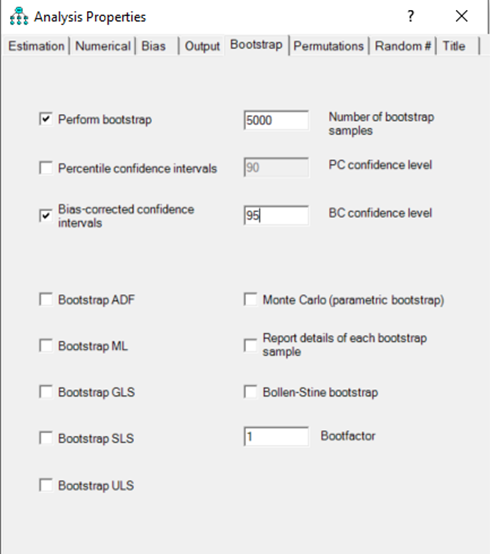## Step 3: Analyzing Mediation Analysis Results

• Let’s look at the output to determine if mediation is present.
• In the Estimates link, you want to select the “Matrices” link. This will let you see the total effects, direct effects, and indirect effects for each relationship in your model.
• We want to select the indirect effects. AMOS will give you the option to examine the unstandardized or standardized indirect effect. With most mediation analyses, you will see the unstandardized indirect effect reported.
• If you were looking to compare indirect effects within a model, you could easily do so with the standardized indirect effects, but normally, the unstandardized indirect effects are reported. In the “Indirect Effects” tab, you will see all the possible indirect effects in your model. We are concerned only with the relationship of Authentic Leadership to Life Satisfaction through Self-Efficacy.
• In our model, we have only one possible mediator from Authentic Leadership to Life Satisafaction, so the indirect effect listed must be through the mediator of Self-Efficacy. (If you have more than one mediator, I will discuss this in another tutorial.

## Indirect Effect - Upper Bound and Lower Bound Confidence Interval

The Indirect effect of Authentic Leadership (AUL_IV) to LS_DV through Self-Efficacy is .268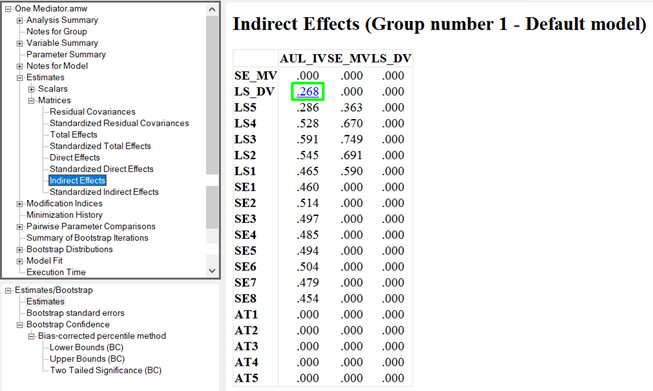• We now know the indirect effect, but we still need to know if the indirect effect is significant and if it falls within the 95% confidence interval generated by our bootstrap. The indirect effects tab will give us the indirect effect but nothing else.
• We need to go to the bootstrap analysis section to find the other information.
• On the left-hand side, in a box below the output links is a section called “Estimates/Bootstrap”. Under that link will be another option called “Bias-corrected percentile method”.
• This is where we will find all the information, we are looking for concerning confidence intervals and significance levels.
• With the confidence intervals, you are going to get an upper bound and lower bound estimate of the indirect effect based on your bootstrap of 5,000 samples.
• If the range for the upper and lower bound estimates do not cross over zero, then the indirect effect is considered significant.
• Following are the results from the Bias-corrected percentile method link.
• It will first present the lower bound indirect effects and then the upper bound indirect effects.
• With our indirect test of Authentic Leadership to Life Satisfaction through Self-Efficacy, the lower bound confidence interval is 0.188 and the upper bound is 0.346.Since there is no zero between the LB and UP CI, this shows significant indirect effects.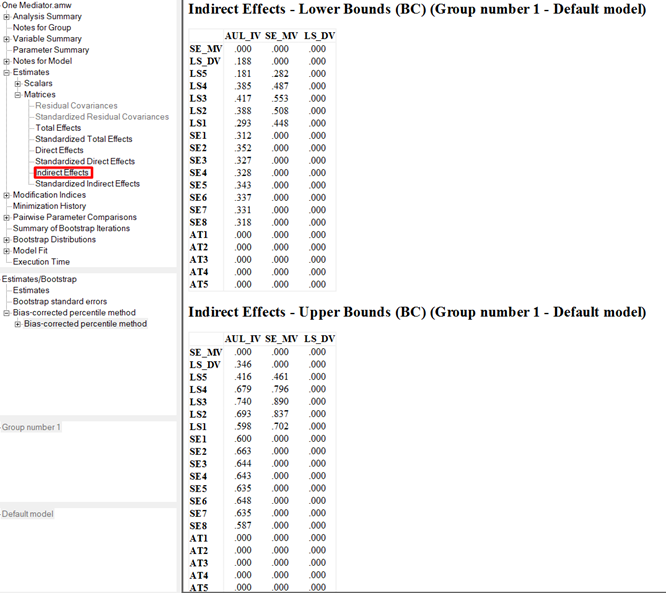## Indirect Effect - Two Tailed Significance

• The values of 0.015 at the intersection of AUL_IV and LS_DV shows the Two Tailed Significance.
• In this case it is .015 which is less than 0.05.
• Hence, we can conclude that Self-efficacy mediates the relationship between Authentic Leadership and Life Satisfaction.